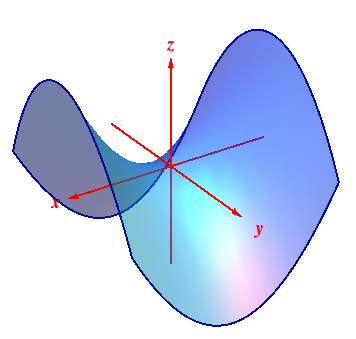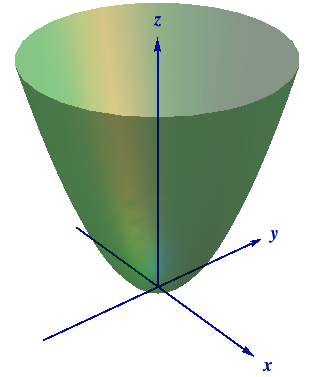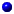M408M Learning Module Pages
Main page

### Chapter 12: Vectors and the Geometry of Space

#### Learning module LM 12.6: Surfaces:

Surfaces and traces
Level curves
Level surfaces
Worked problems

# Surfaces and traces

Surfaces and Traces
Just as having a good understanding of curves in the plane is essential to interpreting the concepts of single variable calculus, so a good understanding of surfaces in $3$-space is needed when developing the fundamental concepts of multi-variable calculus.

We've already seen surfaces like planes, circular cylinders and spheres. The next step is to look at a surface arising as the graph of a real-valued function $z = f(x,\, y) : U \subseteq {\mathbb R}^2 \to {\mathbb R}$ of two variables - in practice, such functions occur in the theory of heat flow in a bar, or vibrating strings, or the pressure at a point on the earth. The graph of $z = f(x,\, y)$ is the surface $$\color{rgb(0, 51, 153)} {S \ = \ \big\{ (x,\, y, \, f(x,\, y)\big) : \, \ (x,\, y) \ \hbox{in} \ U\, \big\} }$$ in $3$-space. Two examples that will occur repeatedly are shown below in$$z \ = \ f(x,\, y) \ = \ x^2 - y^2$$$$\color{rgb(0, 102, 0)}{ z \ = \ f(x,\, y) \ = \ x^2 + y^2}$$

How do we know the surfaces look like that? The basic idea is to take cross-sections of the surface by plane slices. Because a plane intersects the surface in a curve that also lies in the plane, this curve is often referred to as the trace of the surface on the plane. Identifying traces gives us one way of 'picturing' the surface; re-assembling the cross-sections then provides a full picture of the surface. It thus reduces the problem of describing a surface to identfying curves in the plane - the 'natural progression' idea at work! When $z = f(x,\, y)$,the trace on a vertical plane $y=mx+b$ is the curve consisting of all points $$\big\{\, (x,\, mx+b,\, f(x,\, mx+b))\,:\ (x,\, mx+b) \ \hbox{in} \ D\, \big\},$$ in the plane $y = mx + b$,the trace on a horizontal plane $z = c$ is the curve $$\big\{\, (x,\, y,\, c) :\, (x,\, y) \ \, \hbox{in}\, \ D,\ f(x,\,y) = c\ \big\}$$ in the plane $z = c$.

 In the case $z = x^2 - y^2$ slicing vertically by $y = b$ means fixing $y = b$ and graphing $$z \ = \ f(x,\, b) \ = \ x^2 - b^2\,,$$ while slicing vertically by the plane $x = a$ gives $$z \ = \ f(a,\, y) \ = \ a^2 - y^2\,,$$ i.e., parabolas opening up and down respectively. On the other hand, slicing horizontally by $z = c$ gives $$\ f(x,\,y) \ = \ c \ = \ x^2 - y^2\,,$$ i.e., hyperbolas opening in the $x$-direction if $c > 0$ and in the $y$-direction if $c < 0$. So the cross-sections are parabolas or hyperbolas, and the surface is called a hyperbolic paraboloid. You can think of it as a saddle or as a Pringle!

When $z = x^2 + y^2$, the trace on $y = b$ is the graph of $z = x^2+b^2$, while that on $x= a$ is the graph of $z = a^2 + y^2$; these are parabolas which always opening upwards. On the other hand the horizontal trace on $z = c$ is the circle $x^2 + y^2 = c$. So this surface is called a Paraboloid. You can think of it as a crater or a wine-glass!

All this slicing is shown graphically to the left below with the corresponding traces shown to the right: